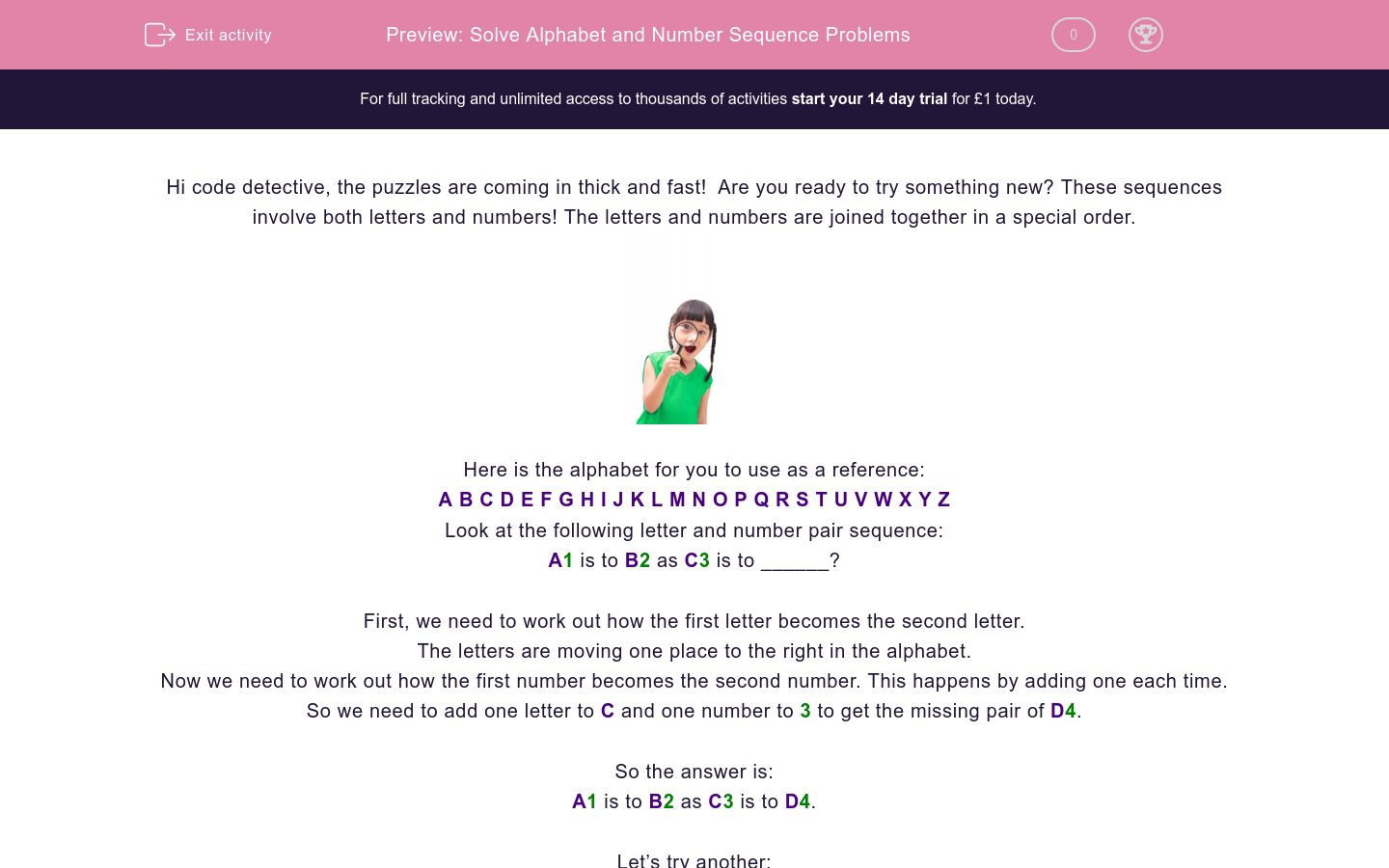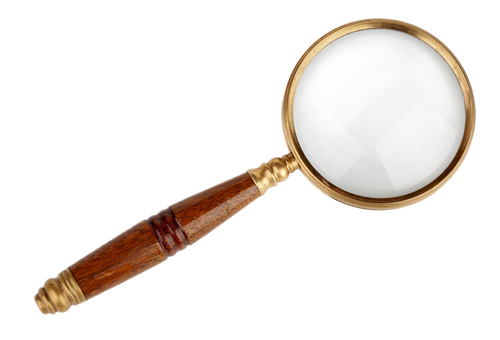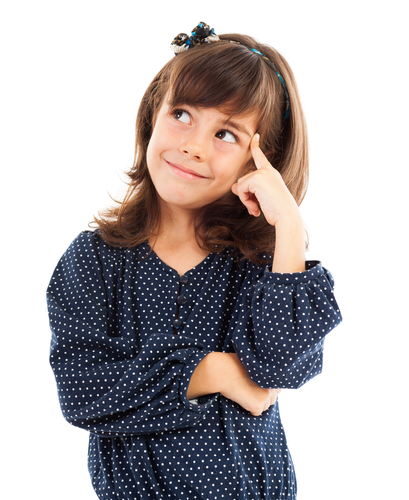# Solve Alphabet and Number Sequence Problems

In this worksheet, students will solve simple sequences involving combined letter and number pairs. It will develop students’ problem solving and sequencing skills.Key stage:  KS 2

Curriculum topic:   Verbal Reasoning

Curriculum subtopic:   Letter Connections

Difficulty level:### QUESTION 1 of 10

Hi code detective, the puzzles are coming in thick and fast!  Are you ready to try something new? These sequences involve both letters and numbers! The letters and numbers are joined together in a special order.Here is the alphabet for you to use as a reference:

A B C D E F G H I J K L M N O P Q R S T U V W X Y Z

Look at the following letter and number pair sequence:

A1 is to B2 as C3 is to ______?

First, we need to work out how the first letter becomes the second letter.

The letters are moving one place to the right in the alphabet.

Now we need to work out how the first number becomes the second number. This happens by adding one each time.

So we need to add one letter to C and one number to 3 to get the missing pair of D4.

A1 is to B2 as C3 is to D4.

Let’s try another:

C4 is to E5 as F7 is to ______?

First, we need to work out how the first letter becomes the second letter.

The letters are moving two places to the right in the alphabet.

Now we need to work out how the first number becomes the second number. This happens by adding one each time.

So we need to add two letters to F and one number to 7 to get the missing pair of H8.

C4 is to E5 as F7 is to H8.

In this activity, you will need to be a sequence superstar to complete the number and letter pairs.

Remember to use the alphabet line to help you work out the code.

Here are two groups of letter and number sequences. Each group follows the same special sequence, but with different letters and numbers.

C1 is to D2 as E3 is to ______ ?A  B  C  D  E  F  G  H  I  J  K  L  M  N  O  P  Q  R  S  T  U  V  W  X  Y  Z

Here are two groups of letter and number sequences. Each group follows the same special sequence, but with different letters and numbers.

G5 is to H6 as L7 is to ______ ?

 A  B  C  D  E  F  G  H  I  J  K  L  M  N  O  P  Q  R  S  T  U  V  W  X  Y  Z

Here are two groups of letter and number sequences. Each group follows the same special sequence, but with different letters and numbers.

A2 is to B4 as C6 is to ______ ?A  B  C  D  E  F  G  H  I  J  K  L  M  N  O  P  Q  R  S  T  U  V  W  X  Y  Z

Here are two groups of letter and number sequences. Each group follows the same special sequence, but with different letters and numbers.

E1 is to G2 as I3 is to ______ ?

 A  B  C  D  E  F  G  H  I  J  K  L  M  N  O  P  Q  R  S  T  U  V  W  X  Y  Z

Here are two groups of letter and number sequences. Each group follows the same special sequence, but with different letters and numbers.

G4 is to H5 as Q7 is to ______ ?A  B  C  D  E  F  G  H  I  J  K  L  M  N  O  P  Q  R  S  T  U  V  W  X  Y  Z

Here are two groups of numbers. Each group follows the same special sequence, but with different numbers.

M5 is to N7 as U15 is to ______ ?A  B  C  D  E  F  G  H  I  J  K  L  M  N  O  P  Q  R  S  T  U  V  W  X  Y  Z

Here are two groups of letter and number sequences. Each group follows the same special sequence, but with different letters and numbers.

U9 is to W10 as E17 is to ______ ?

 A  B  C  D  E  F  G  H  I  J  K  L  M  N  O  P  Q  R  S  T  U  V  W  X  Y  Z

Here are two groups of letter and number sequences. Each group follows the same special sequence, but with different letters and numbers.

W11 is to X14 as G45 is to ______ ?A  B  C  D  E  F  G  H  I  J  K  L  M  N  O  P  Q  R  S  T  U  V  W  X  Y  Z

Here are two groups of numbers. Each group follows the same special sequence, but with different numbers.

K12 is to O13 as E22 is to ______ ?

 A  B  C  D  E  F  G  H  I  J  K  L  M  N  O  P  Q  R  S  T  U  V  W  X  Y  Z

Here are two groups of letter and number sequences. Each group follows the same special sequence, but with different letters and numbers.

D23 is to E28 as H33 is to ______ ?A  B  C  D  E  F  G  H  I  J  K  L  M  N  O  P  Q  R  S  T  U  V  W  X  Y  Z

• Question 1

Here are two groups of letter and number sequences. Each group follows the same special sequence, but with different letters and numbers.

C1 is to D2 as E3 is to ______ ?A  B  C  D  E  F  G  H  I  J  K  L  M  N  O  P  Q  R  S  T  U  V  W  X  Y  Z
F4
EDDIE SAYS
Hi there, detective! The letter sequence here is to follow the alphabet going forwards one jump. The number sequence is to add one.
Top tip: Work out the letter sequence part first, then solve the number sequence part.
• Question 2

Here are two groups of letter and number sequences. Each group follows the same special sequence, but with different letters and numbers.

G5 is to H6 as L7 is to ______ ?

 A  B  C  D  E  F  G  H  I  J  K  L  M  N  O  P  Q  R  S  T  U  V  W  X  Y  Z
M8
EDDIE SAYS
The letter sequence is to make one jump forwards in the alphabet. The number sequence is to add one.
Top tip: Use a piece of paper to do your working out on if you need to!
• Question 3

Here are two groups of letter and number sequences. Each group follows the same special sequence, but with different letters and numbers.

A2 is to B4 as C6 is to ______ ?A  B  C  D  E  F  G  H  I  J  K  L  M  N  O  P  Q  R  S  T  U  V  W  X  Y  Z
D8
EDDIE SAYS
Getting the hang of it? The letter sequence here is to follow the alphabet forwards one jump. Whilst the number sequence is to add two.
• Question 4

Here are two groups of letter and number sequences. Each group follows the same special sequence, but with different letters and numbers.

E1 is to G2 as I3 is to ______ ?

 A  B  C  D  E  F  G  H  I  J  K  L  M  N  O  P  Q  R  S  T  U  V  W  X  Y  Z

K4
EDDIE SAYS
Brilliant effort, detective! The letter sequence is to make two jumps forwards in the alphabet. The number sequence is to add one.
• Question 5

Here are two groups of letter and number sequences. Each group follows the same special sequence, but with different letters and numbers.

G4 is to H5 as Q7 is to ______ ?A  B  C  D  E  F  G  H  I  J  K  L  M  N  O  P  Q  R  S  T  U  V  W  X  Y  Z

R8
EDDIE SAYS
Did you find the missing letter and number? The letter sequence follows the alphabet forwards, with one jump. Meanwhile, the number sequence is to add one.
• Question 6

Here are two groups of numbers. Each group follows the same special sequence, but with different numbers.

M5 is to N7 as U15 is to ______ ?A  B  C  D  E  F  G  H  I  J  K  L  M  N  O  P  Q  R  S  T  U  V  W  X  Y  Z
V17
EDDIE SAYS
Super sequencing skills! The letter sequence is to follow the alphabet with one jump forwards. The number sequence is to add two.
• Question 7

Here are two groups of letter and number sequences. Each group follows the same special sequence, but with different letters and numbers.

U9 is to W10 as E17 is to ______ ?

 A  B  C  D  E  F  G  H  I  J  K  L  M  N  O  P  Q  R  S  T  U  V  W  X  Y  Z
G18
EDDIE SAYS
You're doing a fantastic job, detective! The letter sequence is to count forward two jumps in the alphabet. The number sequence is to add one.
• Question 8

Here are two groups of letter and number sequences. Each group follows the same special sequence, but with different letters and numbers.

W11 is to X14 as G45 is to ______ ?A  B  C  D  E  F  G  H  I  J  K  L  M  N  O  P  Q  R  S  T  U  V  W  X  Y  Z

H48
EDDIE SAYS
What could be better, than finding numbers and letters?! The letter sequence here is to follow the alphabet forwards, making one jump. The number sequence is to add three.
• Question 9

Here are two groups of numbers. Each group follows the same special sequence, but with different numbers.

K12 is to O13 as E22 is to ______ ?

 A  B  C  D  E  F  G  H  I  J  K  L  M  N  O  P  Q  R  S  T  U  V  W  X  Y  Z

I23
EDDIE SAYS
The letter sequence is to make four jumps forwards in the alphabet. The number sequence is to add one. Can you solve the last sequence in this activity? Let's see what it is!
• Question 10

Here are two groups of letter and number sequences. Each group follows the same special sequence, but with different letters and numbers.

D23 is to E28 as H33 is to ______ ?A  B  C  D  E  F  G  H  I  J  K  L  M  N  O  P  Q  R  S  T  U  V  W  X  Y  Z

I38
EDDIE SAYS
The letter sequence is to follow the alphabet forwards, making one jump. The number sequence is to add five.
Shhh, don't make a sound... All the letters and numbers have been found!
---- OR ----

Sign up for a £1 trial so you can track and measure your child's progress on this activity.

### What is EdPlace?

We're your National Curriculum aligned online education content provider helping each child succeed in English, maths and science from year 1 to GCSE. With an EdPlace account you’ll be able to track and measure progress, helping each child achieve their best. We build confidence and attainment by personalising each child’s learning at a level that suits them.

Get started